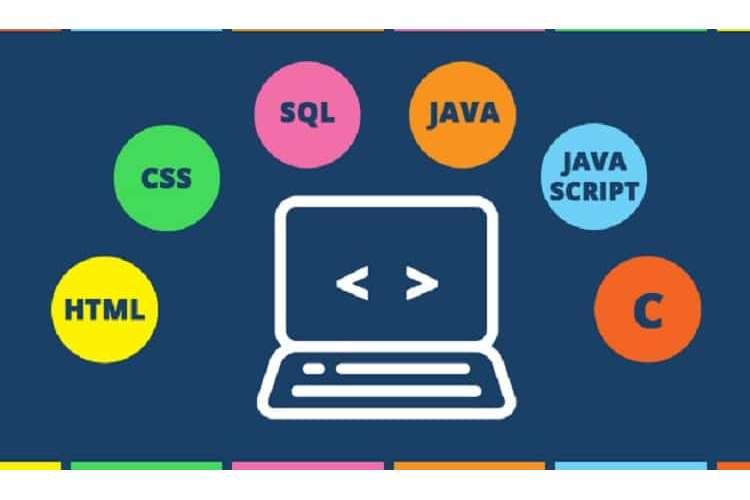## Introduction

Machine learning and data analytics has become an important part of every industry ranging from the FMCG companies to the health care and the logistics industries. Availability of different types of data (both structured and the unstructured data) and the analytical and statistical techniques has helped various industries to make better business decisions(Acharjya & P 2016; Xie et al. 2018) . From predicting the weather to predicting the customer purchasing behavior data analytics has become an important part of every business. So, in the project is also aimed to implement some of the machine learning techniques and interpret the findings. This project will focus majorly on the classification techniques. Some of the classification techniques included in the current project are the random forest, support vector machine, KNN and the logistic regression. All the techniques have been tested on the same data set so that the comparison can be made. The comparison of the models and identification of the best model has been presented in the last part of the project management.

### Task A: Understanding of the data

The first part is focused on the understanding the given data set from various perspective. For analysis, whether it is a big or small, the understanding of the data is very important. Until and unless the data is understood properly, the appropriate analysis cannot be performed. In other words, to find useful information from the given data, understanding of the given data is very important(Ziafat & Shakeri 2014) . In this section, the understanding of the data has been done on the basis of the aim and the number of the data points in the data set.

As the data and the given guidelines suggests, the main objective of the current data analysis project is to use the different analytical and statistical techniques and profile the customers/individual’s behavior. In other words classifying the individuals in different groups based on their behavior.

Data from both the train and test data set shows that there are different kinds of activities included in the research. These are the daily activities of the human beings or more specifically the physical activities. Some of them are walking, lying down, climbing the stairs, sitting etc.

In terms of the data, the total number of rows are 7352, which means there are 7352 instances, whereas the data shows that the number of columns are 561 which are also the number of features. Data also shows that the data was collected among 30 different individuals.

While talking about the results, among the different classification techniques used in the study, the results from the SVM method shows the most promising results with 96 % accuracy.

### Task B: K-Nearest Neighbor Classification

The second part of the research is focused on the implementing the K Nearest Neighbor which is one of the most popular classification techniques. For all the analysis in the current study, the programming language python has been used.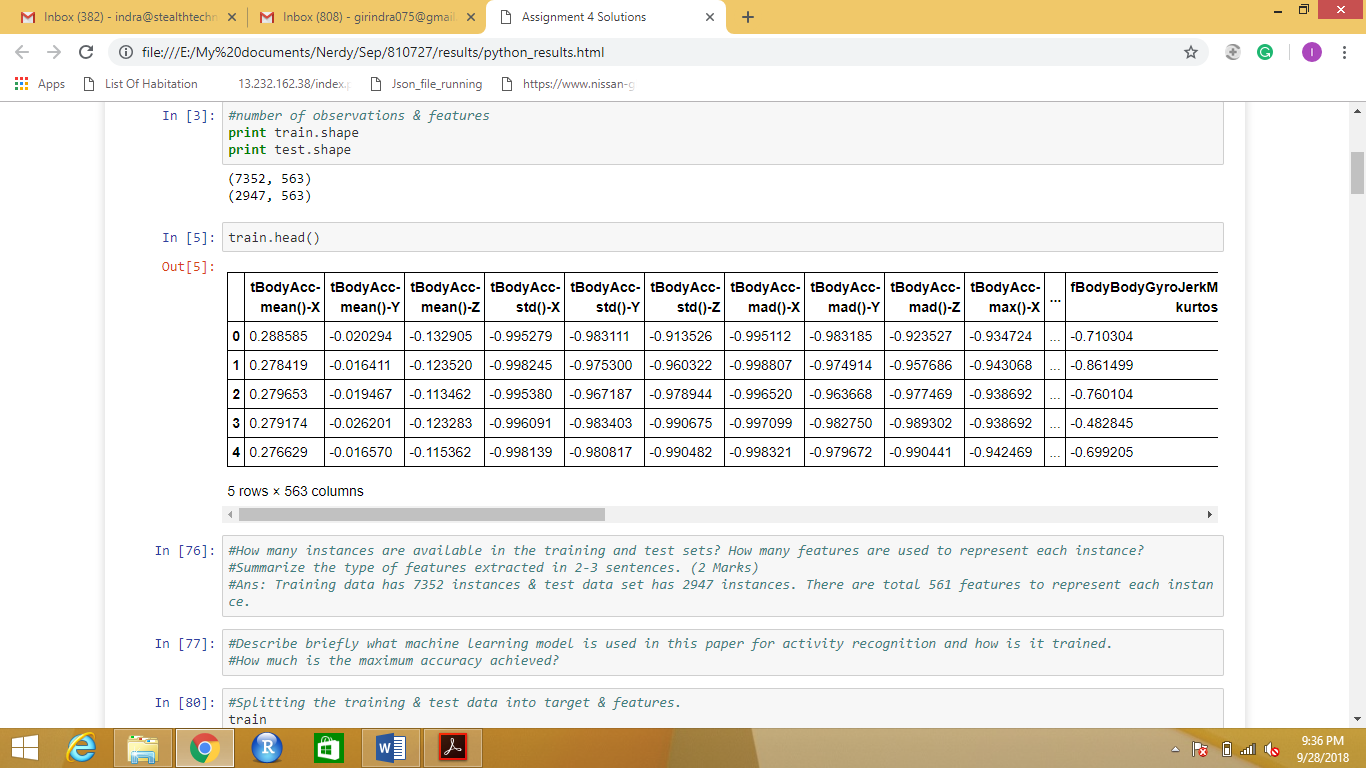Table 1 overview of the data

The overview of the data has been shown in the above figure.

As per the given instruction in the project in the first stage a 10 fold cross validation has been developed. In this cross validation, the minimum value of k is 1 and the maximum value is 50.

The results from this analysis shows that the f1 score (which has been used for the current analysis as the evaluation parameter) is around 0.97 when the training data set was used and when the same was used for the test data it was 0.90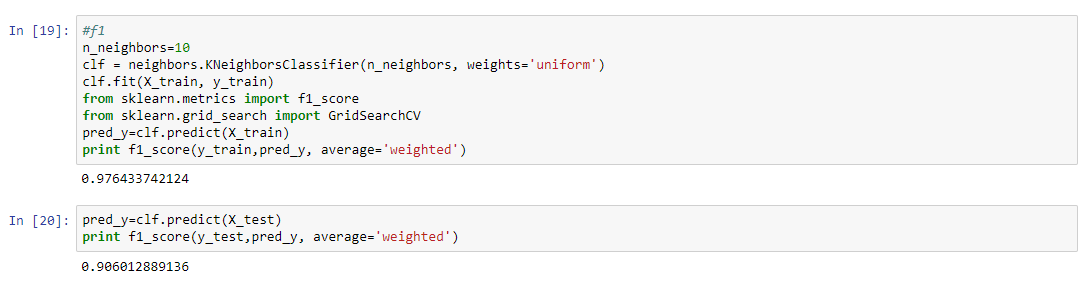Table 2 calculating the f1 score for KNN model

Now, the next step is to run the Gridsearch and the results from the gridsearch is shown below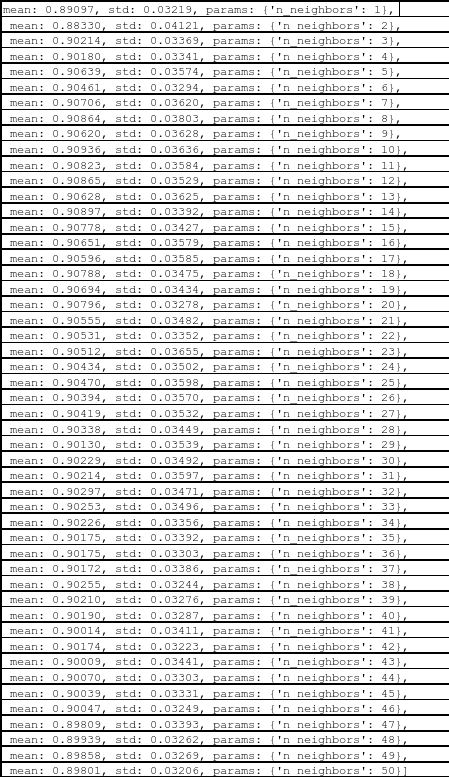Table Results from the gridsearch for KNN model

Now, the next task is to find the best estimator the knn.best.estimator has been used.Now, for all the values from the grid search has been used and the f1 score has been tested for each iteration.

### Cross Validation plot

In this section the results from the cross validation has been discussed and the plot has been show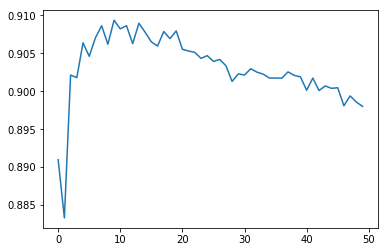### Figure 1Cross validationfor the KNN model

On the basis of the results from the cross validation, the f1 score continue to increase till k = 10. In fact f1 is highest when k = 10. After that the f1 value declines. So, on the basis of this results the accuracy score and the confusion matrix has been constructed.

0.906012889136

0.906684764167

[[534 2 1 0 0 0]

[ 0 409 78 0 0 4]

[ 0 47 485 0 0 0]

[ 0 0 0 486 10 0]

[ 0 0 0 51 331 38]

[ 0 0 0 36 8 427]]

As the results show the f1 value is 0.90 and also the value of accuracy is 0.90. So, it can be concluded that our model is 90 % accurate.

### Task C: Multiclass Logistic Regression with Elastic Net

Another model used in the current project is the Multiple logistics economic model and the results from the model are discussed in the current section (Armstrong 2012; Cerrito 2010; George, Seals & Aban 2014) .

The results from the gridsearch for logistic regresion is shown below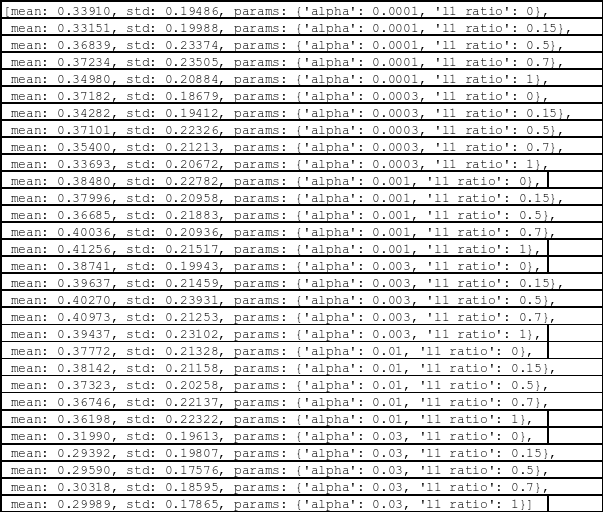Table 3 Results of Grid Search for the logistic regression

On the basis of the gridsearch , the best estimator will be identified. In this case the best alpha value has comes out to be 0.001 when L1_ration takes the value 0.

Now, based on L1_ration and the best alpha value the researcher have plotted the cross validation plot.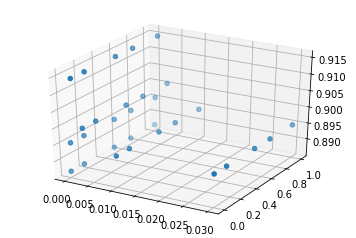Figure 2 Cross validation plot for the logistic regression

On the basis cross validation results, the best alpha has been identified. However this was all done on the train data. Now, the same can be implied on the given test data.

0.916455898484

[[537 0 0 0 0 0]

[ 5 336 145 2 0 3]

[ 0 7 523 2 0 0]

[ 0 0 0 494 2 0]

[ 0 0 0 16 394 10]

[ 0 0 0 46 3 422]]

Similar to the previous case, when the model was run on the test data, the f1 score comes out to be 0.91. In other words the model is 91% accurate. The same for the KNN model was 90 %

### Task D: Support Vector Machine ( RBF Kernal)

In this fourth section another popular classification technique has been discussed and this technique is SVM or Support Vector Machine. In this the RBF kernel will be used (Bhavsar & Panchal 2012) .

In this case the SVM has been optimized on the basis of the following two parameters:

The first one is “C” which is the penalty meter of the error term. Whereas the second is the “Gamma” which is described as the kernel coefficient for the functional form.

In this case also the grisearch has been used for the purpose of tuning the given hyper parameters and the SVM best estimator has been identified. .

Identification of classification of SVM best estimator:

The value of C and Gamma are as follows:

{'gamma': [1e-3,1e-4],

'C':[1,10,100,1000]}

On the basis of the above results, the optimal values are:

C = 1000

gamma value= 0.001

Now, the next step is to plot the cross validation plot on the basis of the given “C” and “Gamma”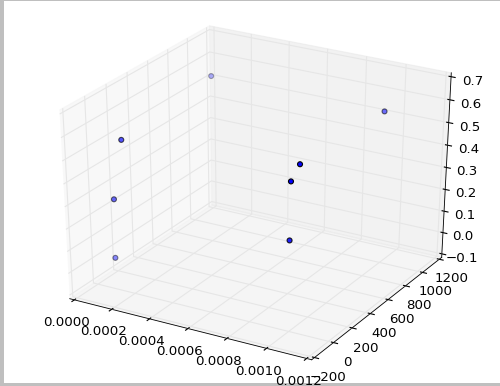Figure 3 Cross validation for the SVM model

After the plot, the next step is to calculate the f1 and cofusion matrix. For SVM the results are as follows:

0.965624534728

[[537 0 0 0 0 0]

[ 0 436 53 0 0 2]

[ 0 12 520 0 0 0]

[ 0 0 0 493 3 0]

[ 0 0 0 4 406 10]

[ 0 0 0 17 0 454]]

As the results shows, the f1 is 0.96 which indicates that there is 96 % accuracy in our model when SVM technique is used. This is highest as compared to the f1 score of previous classifier techniques.

The last technique used in the current case is the random forest which is another popular classification techniques used by the researchers (Biau 2012) .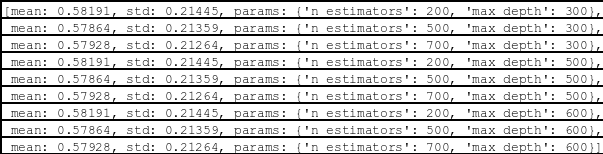Table 4 Results from the GridSearch for random forest

The results of the grid research for Random forest is shown in table above. In this case the tuning of the parameters has been conducted based on the trees numbers and the maximum depth of the tree. To show the results visually the plot has been shown in the figure below with respect to f1.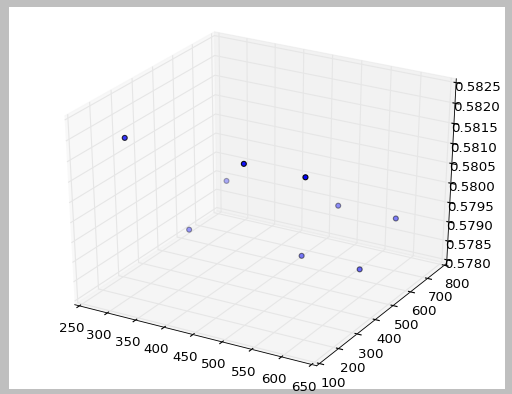Figure 4 Cross validation plot for the random forest

The results for the f1 and confusion matrix for random forest is shown below

0.928839558732

[[537 0 0 0 0 0]

[ 0 441 50 0 0 0]

[ 0 42 490 0 0 0]

[ 0 0 0 482 9 5]

[ 0 0 0 20 357 43]

[ 0 0 0 34 6 431]]

As the results shows the f1 value is 0.92 which indicates that the model is 92 % accurate.

### Task F: Comparison of the different models

Different classification models have been discussed in the current project and for the evaluation purpose the f1 score has been used. There are other parameters also which can be used such as the precision, recall, accuracy. However f1 is considered to be most reliable so f1 has been used in this case. On the basis of the results from f1 in different models, the best model comes out to be the SVM model. This is because the f1 score was highest (96%) in this model. However the results can be different if other parameters are used for the comparison. Also, it should be noted that different types of classifiers have their advantages and limitations. The selection of the model also depends on the types of data and the main aim of the classification.

#### References:

1. Acharjya, DP & P, KA 2016, ‘A Survey on Big Data Analytics: Challenges, Open Research Issues and Tools’, International Journal of Advanced Computer Science and Applications,, vol. 7, no. 2, pp. 511–518.
2. Armstrong, JS 2012, ‘Illusions in regression analysis’, International Journal of Forecasting,, vol. 6, pp. 689–694.
3. Bhavsar, H & Panchal, MH 2012, ‘A Review on Support Vector Machine for Data Classification’, International Journal of Advanced Research in Computer Engineering & Technology, vol. 1, no. 10, pp. 185–189.
4. Biau, G 2012, ‘Analysis of a Random Forests Model’, Journal of Machine Learning Research, vol. 13, pp. 1063–1095.
5. Cerrito, PB 2010, The Difference Between Predictive Modeling and Regression, Louisville.
6. George, B, Seals, S & Aban, I 2014, ‘Survival system analysisand regression models’, NCBI, vol. 21, no. 4, pp. 686–694.
7. Xie, Ji, Song, Z, Li, Y, Zhang, Y, Yu, H, Zhan, J, Ma, Z, Qiao, Y, Zhang, J & Guo, J 2018, ‘A Survey on Machine Learning-Based Mobile Big Data Analysis: Challenges and Applications’, Wireless Communications and Mobile Computing, vol. 2018, pp. 1–19.
8. Ziafat, H & Shakeri, M 2014, ‘Using Data Mining Techniques in Customer Segmentation’, Int. Journal of Engineering Research and Applications, vol. 4, no. 9, pp. 70–79